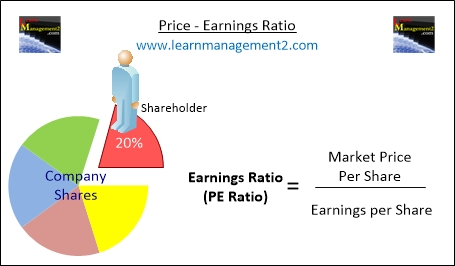# Price-Earnings Ratio (P/E Ratio)

People that own a business are called shareholders because they own part (or share) of a business. Anybody that buys shares in a business is known as a shareholder. Shareholders are interested in how much money (return) the firm will make for them. Shareholder ratios calculate the return shareholders receive from the money they have invested in a firm; in this article we will discuss the shareholder ratio called The Earnings Ratio (price/earnings ratio, sometimes referred to as P/E ratio or PER).

Click to learn about the Earnings Per Share Ratio and The Dividend Yield, the other shareholder ratios.This diagram show how to calculate the Price-Earnings Ratio

### What Is The Price - Earnings Ratio

The Price - Earnings Ratio (also known as the PE ratio) analyses the firm's current share price against how much money each share is generating. This gives investors an idea about the likely returns (money) they could make if they decided to buy shares in the firm.

### How To Calculate The Price - Earnings Ratio

To calculate The Price - Earnings Ratio find out

• The market price per share and
• The earnings per share

Divide the market price per share by the earnings made by share.

Price - Earnings Ratio            Market Price per Share (pence/cents)
=          _________________

Earnings per Share (pence/cents)

### Example Calculation

For example if a firm’s market price per share is £10 and the earnings per share is 50p, the earnings ratio would be calculated as follows:

Price -Earnings                        1000
Ratio                        =          ______            =          20

50

### What Does the Answer (Earnings Ratio) You Get Mean?

The Price - Earnings ratio shows how many times the firm’s current share price is bigger than the earnings each ordinary share generates; or in other words how many times the firm’s current share price is bigger than earnings per share. Another way to describe the Price - Earnings ratio is that it shows how much money you need to spend on shares to make one pound/dollar from the shares you buy.

### Will A Firm Always Have The Same Price-Earnings Ratio?

The Price - Earnings ratio will change as and when the market price for the firm’s shares changes. The number of times that the firm’s share market price changes (throughout the year) will depend on the firm, economic conditions and the industry that the firm is based in.

We hope you have enjoyed learning about the Price - Earnings Ratio, if you would like to learn more, click to learn about the Earnings Per Share Ratio and The Dividend Yield the other shareholder ratios.